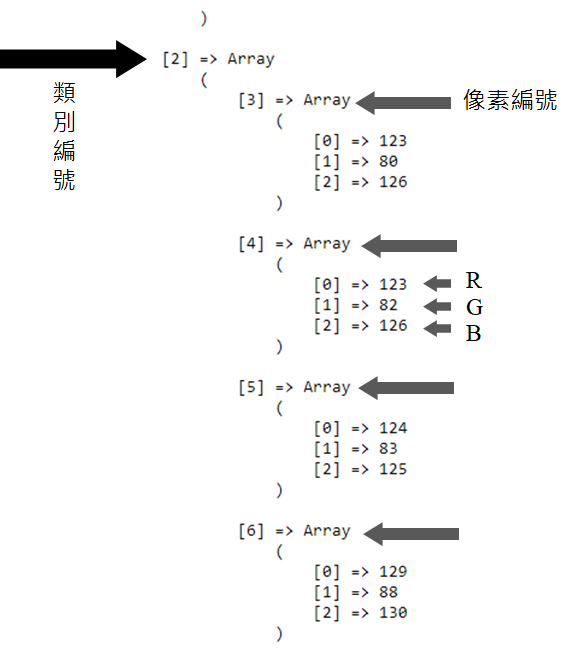#DAY 15
0
AI & Data

## 今日Code簡易解說

K-means函式分類

``````\$kmeans = new KMeans(3);
\$result = \$kmeans->cluster(\$kmeansRGBSample);
``````

## 完整範例Code

``````<?php
//執行時間上限的部份單位是秒，如果要設為無上限可以設定0
set_time_limit(0);

use Phpml\Clustering\KMeans;

\$source_file = "test_image.jpg";

\$im = ImageCreateFromJpeg(\$source_file);
\$imgw = imagesx(\$im);
\$imgh = imagesy(\$im);
\$newFilename = md5(date("YmdHis")).".jpg";

//宣告K-means RGB Sample
\$kmeansRGBSample = array();

for (\$i=0; \$i<\$imgw; \$i++)
{
for (\$j=0; \$j<\$imgh; \$j++)
{
// 取得當前像素的RGB值
\$rgb = ImageColorAt(\$im, \$i, \$j);

// 取得RGB的各別值
\$image_R = (\$rgb >> 16) & 0xFF;
\$image_G = (\$rgb >> 8) & 0xFF;
\$image_B = \$rgb & 0xFF;

\$kmeansRGBSample[] = [\$image_R, \$image_G, \$image_B];

// 印出RGB各別值
// echo "R:\$image_R, G:\$image_G, B:\$image_B
";
}
}

\$kmeans = new KMeans(3);
\$result = \$kmeans->cluster(\$kmeansRGBSample);

echo "<pre>";
print_r(\$result);
echo "</pre>";

// 輸出圖像到文件
// imagejpeg(\$im, "./images/\$newFilename");
?>
``````